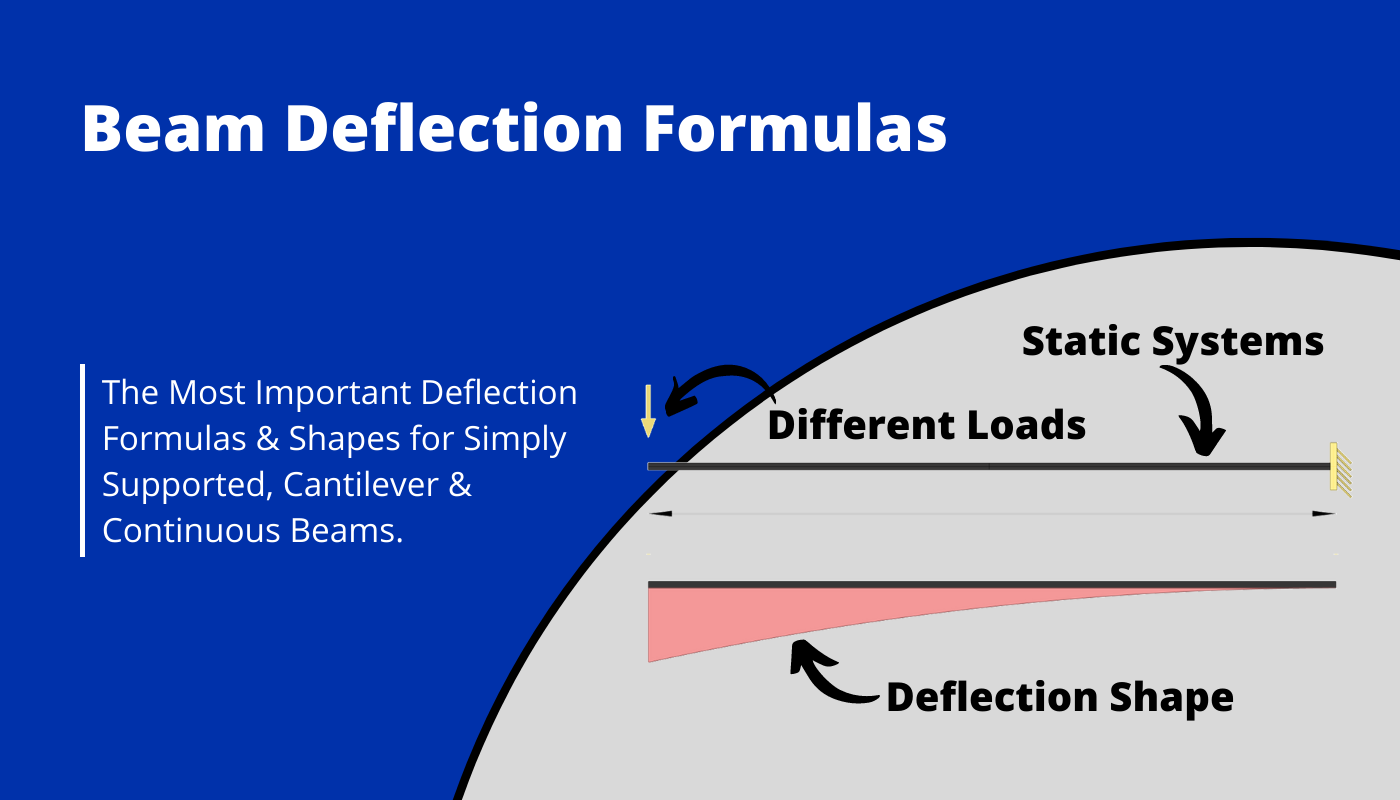# 13 Beam Deflection FormulasLast updated: June 6th, 2023

Calculating reaction forces, internal forces and deflections of beams for different loading scenarios, is one of the things in structural engineering that we do throughout our studies and also careers later on.

While it’s very important to know how to calculate reaction and internal forces, it’s much more difficult to calculate the deflection of beams due to different loads.

In case it’s not a simply supported beam, you most likely have to either look up the formula from a book or use an advanced FEM program.

Now, before we get started, the unit of the deflection is in most cases [mm] for most European countries.

But now, let’s jump into it.

## Simply Supported Beam: Uniformly Distributed Line Load

This is the most used static system and deflection formulas🔥.

### Line Load | Simply Supported Beam

#### Max. Deflection $w_{max}$

$w_{max} = \frac{5}{384} \cdot \frac{ql^4}{EI}$

## Simply Supported Beam: Point Load on Midspan

### Point Load Midspan | Simply Supported Beam

#### Max. Deflection $w_{max}$

$w_{max} = \frac{Ql^3}{48EI}$

## Simply Supported Beam: Intermediate Point Load

### Intermediate Point Load | Simply Supported Beam

#### Max. Deflection $w_{max}$

$w_{max} = \frac{Qa\cdot(l^2-a^2)^{3/2}}{9l\cdot \sqrt{3}\cdot EI}$ if a<b

## Simply Supported Beam: Moment on 1 End

### 1 External Moment | Simply Supported Beam

#### Max. Deflection $w_{max}$

$w_{max} = \frac{M\cdot l^2}{9\cdot \sqrt{3}\cdot EI}$

## Simply Supported Beam: 2 Point Loads

### 2 Point Loads | Simply Supported Beam

#### Max. Deflection $w_{max}$

$w_{max} = \frac{F\cdot a}{24\cdot EI} \cdot (3l^2-4a^2)$

## Cantilever Beam: Uniformly Distributed Line Load (UDL)

### Line Load | Cantilever Beam

#### Max. Deflection $w_{max}$

$w_{max} = \frac{ql^4}{8\cdot EI}$

## Cantilever Beam: Point Load at Free End

### Point Load – Free End | Cantilever Beam

#### Max. Deflection $w_{max}$

$w_{max} = \frac{Ql^3}{3\cdot EI}$

## Cantilever Beam: Intermediate Point Load

### Intermediate Point Load | Cantilever Beam

#### Max. Deflection $w_{max}$

$w_{max} = \frac{Qa^3}{6\cdot EI}\cdot (3l-a)$

## 2-Span Continuous Beam: Uniformly Distributed Line Load

### UDL Line Load | Continuous Beam Deflection

#### Max. Deflection $w_{max}$

$w_{ab} = 0.00521 \frac{ql^4}{EI}$

$w_{bc} = 0.00521 \frac{ql^4}{EI}$

E = E-modulus of the Beam Material

I = Moment of Inertia of Beam

## 2-Span Continuous Beam: Uniformly Distributed Line Load on 1 span

### UDL Line Load on 1 span | Continuous Beam

#### Max. Deflection $w_{max}$

$w_{ab} = 0.00911 \frac{ql^4}{EI}$

$w_{bc} = -0.00391 \frac{ql^4}{EI}$

E = E-modulus of the Beam Material

I = Moment of Inertia of Beam

## 2-Span Continuous Beam: Point Load on 1 span

### Point Load on 1 span | Continuous Beam

#### Max. Deflection $w_{max}$

$w_{ab} = -0.00588 \frac{Ql^3}{EI}$

$w_{bc} = 0.01495 \frac{ql^4}{EI}$

E = E-modulus of the Beam Material

I = Moment of Inertia of Beam

## 3-Span Continuous Beam: Uniformly Distributed Line Load

### UDL Line Load | Continuous Beam Deflection

#### Max. Deflection $w_{max}$

$w_{ab} = w_{cd} = 0.00677 \frac{ql^4}{EI}$

$w_{bc} = 0.00052 \frac{ql^4}{EI}$

E = E-modulus of the Beam Material

I = Moment of Inertia of Beam

## 3-Span Continuous Beam: Uniformly Distributed Line Load on Center Span

### UDL Line Load on Center | Continuous Beam Deflection

#### Max. Deflection $w_{max}$

$w_{ab} = w_{cd} = -0.00313 \frac{ql^4}{EI}$

$w_{bc} = 0.00677 \frac{ql^4}{EI}$

E = E-modulus of the Beam Material

I = Moment of Inertia of Beam

If you are new to structural design, then check out our design tutorials where you can learn how to use the deflection of beams to design structural elements such as

Do you miss any deflection formulas for beams that we forgot in this article? Let us know in the comments✍️